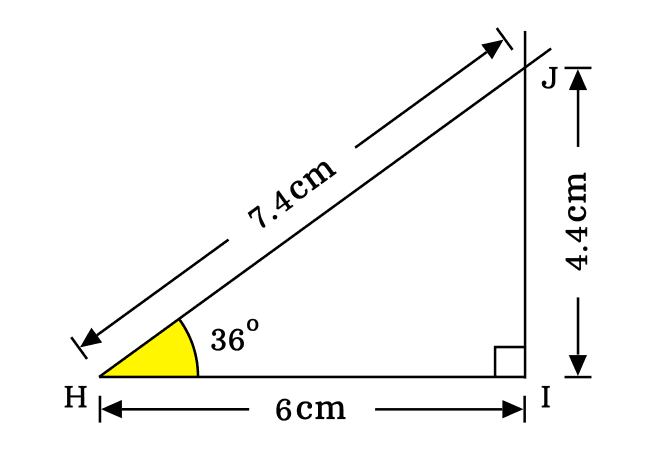# Proof of $\sin{(36^°)}$ value

The exact value of sin 36 degrees in fraction is equal to the quotient of square root of ten minus two times square root of five by four.

$\sin{(36^°)}$ $\,=\,$ $\dfrac{\sqrt{10-2\sqrt{5}}}{4}$

The sine of angle pi by five radian can be derived in two distinct methods in mathematics. Therefore, let’s learn both methods to derive the sine of angle forty grades value mathematically.

## Trigonometric method

Actually, it is not possible to derive the sine of angle thirty six degrees value directly from the trigonometric fundamentals but it can be derived on the basis of the cos 36 degrees value by a trigonometric identity.

#### Express the Sine in Cosine function

As we know that the sine and cosine functions have mutual relationship in the form of a Pythagorean identity. Hence, the sine of angle thirty six degrees can be written in terms of cosine of angle thirty six degrees in mathematical form.

$\sin^2{(36^°)}+\cos^2{(36^°)}$ $\,=\,$ $1$

$\implies$ $\sin^2{(36^°)}$ $\,=\,$ $1-\cos^2{(36^°)}$

$\implies$ $\sin{(36^°)}$ $\,=\,$ $\pm \sqrt{1-\cos^2{(36^°)}}$

Now, substitute the cosine of pi by five value in the above mathematical equation for calculating the sine of angle thirty six degrees value exactly.

$\implies$ $\sin{(36^°)}$ $\,=\,$ $\pm \sqrt{1-\Bigg(\dfrac{\sqrt{5}+1}{4}\Bigg)^2}$

#### Simplify the Trigonometric equation

The value of sine of angle thirty six degrees is defined in irrational form and we have to simplify this expression for evaluating the exact value of sine of angle thirty six degrees in trigonometry.

The simplification process can be initiated by the power rule of a quotient.

$\implies$ $\sin{(36^°)}$ $\,=\,$ $\pm \sqrt{1-\dfrac{(\sqrt{5}+1)^2}{4^2}}$

Inside the square root, the square of sum of two terms can be expanded for simplifying the right hand side expression of the equation.

$\implies$ $\sin{(36^°)}$ $\,=\,$ $\pm \sqrt{1-\dfrac{(\sqrt{5})^2+(1)^2+2 \times (\sqrt{5}) \times 1}{16}}$

$\implies$ $\sin{(36^°)}$ $\,=\,$ $\pm \sqrt{1-\dfrac{5+1+2\sqrt{5}}{16}}$

$\implies$ $\sin{(36^°)}$ $\,=\,$ $\pm \sqrt{1-\dfrac{6+2\sqrt{5}}{16}}$

The right hand side expression of the equation can be simplified by the subtraction rule of fractions.

$\implies$ $\sin{(36^°)}$ $\,=\,$ $\pm \sqrt{\dfrac{1 \times 16-(6+2\sqrt{5})}{16}}$

$\implies$ $\sin{(36^°)}$ $\,=\,$ $\pm \sqrt{\dfrac{16-(6+2\sqrt{5})}{16}}$

$\implies$ $\sin{(36^°)}$ $\,=\,$ $\pm \sqrt{\dfrac{16-6-2\sqrt{5}}{16}}$

$\implies$ $\sin{(36^°)}$ $\,=\,$ $\pm \sqrt{\dfrac{10-2\sqrt{5}}{16}}$

$\implies$ $\sin{(36^°)}$ $\,=\,$ $\pm \dfrac{\sqrt{10-2\sqrt{5}}}{4}$

#### Evaluate the Sine of 36 degrees value

The trigonometric procedure has given two values for sine of angle forty grades.

$(1).\,\,\,$ $\sin{(36^°)}$ $\,=\,$ $+\dfrac{\sqrt{10-2\sqrt{5}}}{4}$

$(2).\,\,\,$ $\sin{(36^°)}$ $\,=\,$ $-\dfrac{\sqrt{10-2\sqrt{5}}}{4}$

Actually, The sine function does not give two values for one angle. So, one of them is correct and remaining one is incorrect. Now, it is time to evaluate the exact value of sine of angle pi by five radian.

The angle $36^°$ belongs to the first quadrant in two dimensional Cartesian coordinate system. We know that the sign of sine is positive in the first quadrant.

$\,\,\,\therefore\,\,\,\,\,\,$ $\sin{(36^°)}$ $\,=\,$ $\dfrac{\sqrt{10-2\sqrt{5}}}{4}$

In this way, the sin of thirty six degrees value is derived in fraction form exactly in trigonometry.

### Geometric method

Geometrically, the sine of angle thirty six degrees value can also be derived by constructing a right triangle with an angle thirty six degrees but it is impossible to evaluate the exact value of sin pi by five value due to the problem in measuring the lengths of the sides. However, its value can be derived approximately.1. Draw a line horizontally by a ruler. You can draw it with any length. In this example, a horizontal line is drawn with $6\,cm$ length from the point $H$ to $I$.
2. Set the center (or centre) of protractor to coincide with point $H$ and also coincide its right side base line with horizontal line. Later, draw a straight line with thirty six degrees from point $H$.
3. Now, draw a perpendicular line to horizontal line in top side direction from point $I$ and it intersects the thirty six degrees line at point $J$.

According to the above three steps, a right angled triangle (or right triangle) can be constructed geometrically with an angle of pi by five radian. Now, we can start the mathematical procedure to evaluate the sine of angle thirty six degrees value.

On the basis of $\Delta JHI$, the sine of angle thirty six degrees can be expressed in ratio form of the sides as per its fundamental definition. In this triangle, the opposite side and hypotenuse are $\overline{JI}$ and $\overline{HJ}$ respectively.

$\sin{(36^°)}$ $\,=\,$ $\dfrac{JI}{HJ}$Actually, the lengths of opposite side and hypotenuse are unknown. So, it is not possible to evaluate the sine of forty grades value mathematically. Hence, measure the lengths of them by a ruler. It is measured that the length of opposite side is $4.4\,cm$ and hypotenuse is $7.4\,cm$.

$\implies$ $\sin{(36^°)}$ $\,=\,$ $\dfrac{4.4}{7.4}$

$\implies$ $\sin{(36^°)}$ $\,=\,$ $0.5945945945\cdots$

$\,\,\,\therefore\,\,\,\,\,\,$ $\sin{(36^°)}$ $\,\approx\,$ $0.5946$

##### Conclusion

As per the trigonometric method, the sine of angle forty grades is derived in fraction form as follows.

$\sin{(36^°)}$ $\,=\,$ $\dfrac{\sqrt{10-2\sqrt{5}}}{4}$

$\implies$ $\sin{(36^°)}$ $\,=\,$ $0.5877852522\cdots$

$\implies$ $\sin{(36^°)}$ $\,\approx\,\,$ $0.5878$

As per the geometrical approach, the sine of angle pi by five radian is derived in decimal form as follows.

$\sin{(36^°)}$ $\,\approx\,$ $0.5946$

The sine of thirty six degrees is $0.5878$ in trigonometric method but its value is $0.5946$ in geometrical approach.

$0.5878-0.5946 \,=\, -0.0068$

$\implies$ $0.5878-0.5946 \,\approx\, 0$

The difference between the values of sine of angle pi by five is $-0.0068$, which is approximately zero. However, we cannot consider the value of sine thirty six degrees, obtained from the geometrical method as its exact value because it is calculated by measuring the lengths of opposite side and hypotenuse approximately.

However, the $\sin{\Big(\dfrac{\pi}{5}\Big)}$ value is derived from a trigonometric identity in trigonometric method. Hence, we should take the value of sine of forty grades, obtained from trigonometric approach as the the exact value for the sine of angle thirty six degrees in mathematics.

Latest Math Topics
Email subscription
Math Doubts is a best place to learn mathematics and from basics to advanced scientific level for students, teachers and researchers. Know more